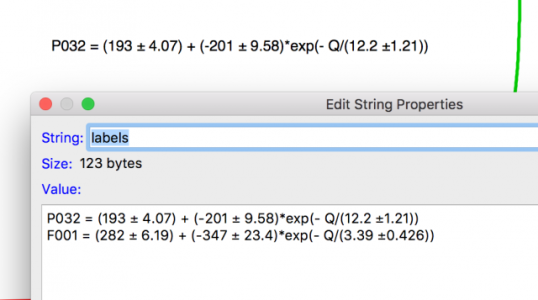# Annotation Text with multiple lines.Hi,

I am trying automate an analysis. In the loop, I do multiple curve fits and record the values in a string for latter annotation.

For example I have a string, called labels, that is two lines and looks like this

P032 = (193 ± 4.07) + (-201 ± 9.58)*exp(- Q/(12.2 ±1.21))
F001 = (282 ± 6.19) + (-347 ± 23.4)*exp(- Q/(3.39 ±0.426))

if I create an annotation using dynamic text \{labels} only the first line of the text is show in the annotation.

P032 = (193 ± 4.07) + (-201 ± 9.58)*exp(- Q/(12.2 ±1.21))

How do I get both (all) lines of the text string to show up?

AndyA simple way is to use two dynamic escape codes, as in
•mylabel1="P032 = (193 ± 4.07) + (-201 ± 9.58)*exp(- Q/(12.2 ±1.21))"
•mylabel2="F001 = (282 ± 6.19) + (-347 ± 23.4)*exp(- Q/(3.39 ±0.426))"

TextBox/C/N=text0/A=MC "\\{mylabel1}\r\\{mylabel2}"

I tried using various \r\n new line escape codes in a single dynamic string without success.
Three things:

1) did you try TextBox ... "something\\r next line"?